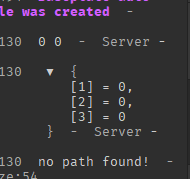# Recursive backtracker help needed

Hello, trying to make use of a maze creation algorithm called the recursive backtracker.
PS: The original code was made in python (so not by me.) and i need help fixing it so it works.

``````
local function valid(i,j)
return (i >= 0 and i <=2) and (j >= 0 and j <= 2)
end

local matrix = { = {'.', '.', '.'},  = {'.', 'X', '.'},  = {'.', 'G', 'X'}}
-- an array that marks indices as visited or not
local visit = { = {0,0,0}, = {0,0,0}, = {0,0,0}}
-- the path that is used in the back tracking
local path = {}
-- this will be a list of lists containing ALL the paths that can reach the GOAL
local ans = {}
-- these are the neighbors indices
local row = {-1, 1, 0, 0}
local col = {0, 0, 1, -1}

local function backtrack(i,j)
-- if matrix[i][j] is visited, then do nothing
-- if it is a wall, then do not insert it in the path from the first place
print(i,j)
print(visit[i])
if visit[i][j] ~= 0 or matrix[i][j] == 'X' then
return
end
-- mark the index as visited as to not visit it again
visit[i][j] = 1
-- add the index to the path
table.insert(path,table.unpack({i,j}))
-- if it is the GOAL, then store this path somewhere,
-- and pop the last index to traverse other paths that reaches the GOAL
if matrix[i][j] == 'G' then
-- any paths that reach the GOAL are appended here
table.insert(ans,table.pack(path))
return
end
-- loop on the neighbors. if they are valid indices, do the same work again
for k = 1,3,1 do
local nwi = i + row[k]
local nwj = j + col[k]
if valid(nwi, nwj) then
backtrack(nwi, nwj)
-- after finishing this index, just pop because we do not need it any more
end
end
return
end

backtrack(0,0)

if #ans == 0 then
print("no path found!")
else
print(ans)
end
``````

Orginal code ^^
So, basically it errors at the fifth line in the function, attempt to index nil with number. Any help is appreciated :))))

What is the output of these lines:

``````print(i,j)
print(visit[i])
``````

You may also wish to add: `print(matrix[i])`Ah.
Try replacing 0,0 with 1,1

In Lua / Luau, arrays start at 1, instead of 0!

that would break it, instead i just forcefully set the indexes in the tables as you can see, i tried what you said earlier and it always ended to it trying to index with 1, so i couldn’t. this works better than before

Just go through it and ensure that no values within square brackets (`matrix[1,0]` would have 1, and 0 in the brackets) are equal to 0!

I won’t have to do this if i just start the tables with 0, :))